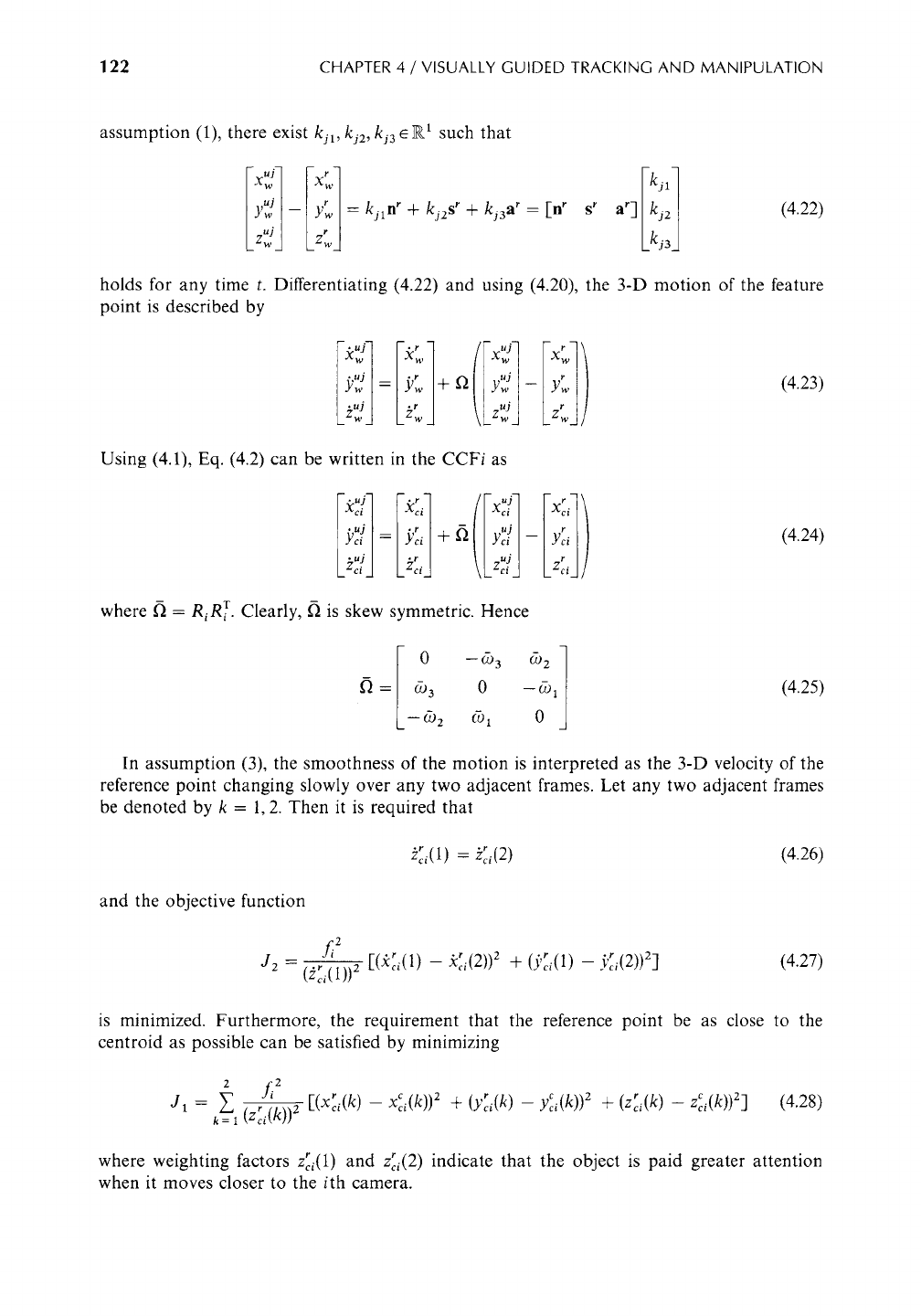3 ESTIMATION OF THE MOTION FIELD OF THE REFERENCE POINT 121
FIGURE 4.2
Motion of a rigid object.
/
!
t r
r t
a , n
~'"'"/:i
s r
!
!
/
!
i !
!
i
zw
Xw< ;
Yw
A reference point of a moving object is defined as a 3-D point such that
1. Its position relative to the object does not change over time.
2. The point has a purely translational motion and no rotation.
3. With the constraint that the translational motion of the reference point is smooth, its
distance from the centroid of the object is kept as small as possible.
The definition of the reference point consists of two important aspects. First, the choice of
the reference point should be such that its motion is smooth and therefore predictable.
Second, because the tracking and grasping will be designed with respect to the reference point,
the point should be kept close to the centroid of the object in order to achieve stable grasping.
The rotational motion of the object can be described as follows. Let rffs rar be a coordinate
frame attached to the object centered at the reference point with each axis being parallel to
the corresponding sides of the hexahedral object (Figure 4.2). When the object moves, the
orientation of the coordinate frame changes (with respect to the WCF) according to (see also
[163)
with
[fir gr ar-] __f~[ff s r a r] (4.20)
0 --(93 (92
~'~ -- (93 0 --(91
(4.21)
--092 (91 0
where co
= [(91 (92 0-)3] T
is the angular velocity.
3 ESTIMATION OF THE MOTION FIELD OF THE REFERENCE POINT
3.1 Formulation of the 3-D Reference Point Problem
The three assumptions that a reference point should satisfy can be described mathematically
as follows. Let (x~, y~, z~) (j = 1, 2,..., or be the coordinates of 3-D feature points in the
objects in the WCF. We assume these points can be observed by each camera. Since rnrsra r
r r r T
is the coordinate frame whose origin is at the reference point xr= [Xw
Yw Zw],
by122 CHAPTER 4 /VISUALLY GUIDED TRACKING AND MANIPULATION
assumption (1), there exist
kjl, kj2, kj3ER 1
such that
Xw Xw kjl
_ZUwJJ Z w kj3
(4.22)
holds for any time t. Differentiating (4.22) and using (4.20), the 3-D motion of the feature
point is described by
i u;] F]
Xw Xw XUw j X w
9
9 uj
LzwJ LzT]
Zw
(4.23)
Using (4.1), Eq. (4.2) can be written in the CCFi as
Xci
where ~ =
RiR ~.
Clearly, ~ is skew symmetric. Hence
0
--(~)3 (~-)2
~'~ -- (-793 0 --(J)l
-- (-~)2 (-~)1 0
(4.25)
In assumption (3), the smoothness of the motion is interpreted as the 3-D velocity of the
reference point changing slowly over any two adjacent frames. Let any two adjacent frames
be denoted by k = 1, 2. Then it is required that
,~i(1) = ,~,(2) (4.26)
and the objective function
f/
2
*~" *r .r .r
J2 -(zci(1))2
[(Xci(1) -- xc,(2)) z +
(y~i(1) -- vc/(2)) 2]
(4.27)
is minimized. Furthermore, the requirement that the reference point be as close to the
centroid as possible can be satisfied by minimizing
L 2
= ~ ~ " Zc,(k)) ]
y~(k)) + (Zci(k) -
(4.28)
J1 k=x (z~/~)) 2 [(Xc~(k) -
x~,(k)) 2 + (yci(k) - ~ 2 ~ ~ 2
where weighting factors z~i(1) and z~i(2) indicate that the object is paid greater attention
when it moves closer to the i th camera.

Get Control in Robotics and Automation now with the O’Reilly learning platform.

O’Reilly members experience books, live events, courses curated by job role, and more from O’Reilly and nearly 200 top publishers.